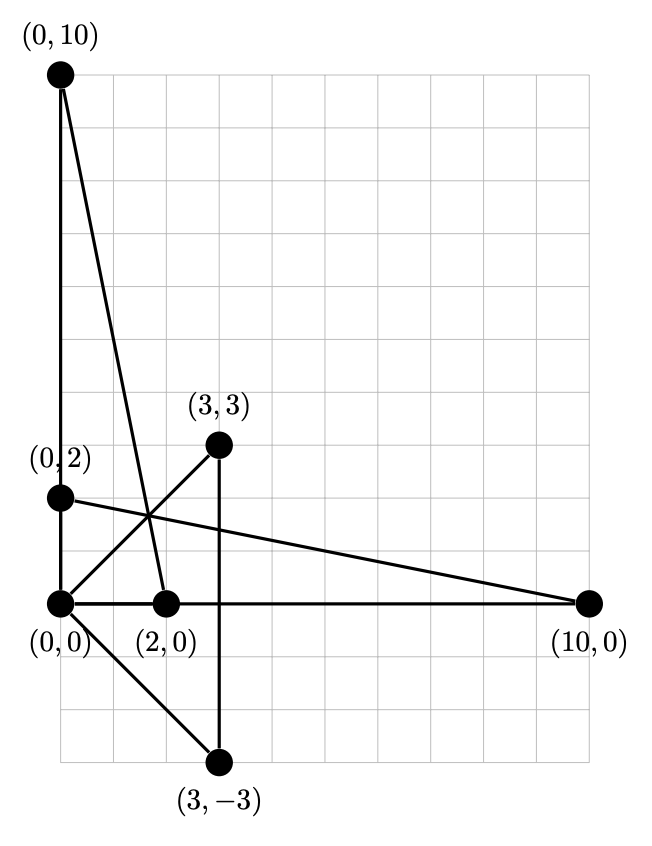시간 제한 메모리 제한 제출 정답 맞은 사람 정답 비율
10 초 256 MB 2 2 2 100.000%

## 문제

$n$ points are given on a plane. We are interested in the number of right-angled triangles with vertices at these points and area contained in the range $[A,B]$.

## 입력

The first line contains three integers $n$, $A$, $B$ ($1\leq n\leq 2000$, $1\leq A\leq B\leq 10^{18}$). The following $n$ lines describe the individual points. The $i$-th of these lines contains two integers $x_i,y_i$ ($-10^9\leq x_i,y_i\leq 10^9$) which are the coordinates of the $i$-th point. All the given points are distinct.

## 출력

The only line of the output should contain the number of triangles with vertices at the given points and area in the range $[A,B]$.

## 예제 입력 1

7 5 25
0 0
2 0
0 2
10 0
0 10
3 3
3 -3


## 예제 출력 1

3


## 힌트